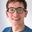## Accrue interest on positive deposit balances every 15 days/half month (French ’intérêts par quinzaine’ method)

What

Accrue interest every 15 days/half month (1st to 15th, and 16th to End Of Month, i.e. 28th, 29th, 30th, or 31st), called the French ‘quinzaine’ process.

The application frequency could be at a different interval, however, and this request concerns accrual frequency only, not application.

For example, with an interest rate of 1.5% per year of 365 days, and interest applied every year (on the 1st of January):

• 10.10.18 deposit of \$5000 made
• Accrual 16.10 to 31.10: 5000*(1.5%/365)*16 = \$3.29
• 12.11.18 deposit of \$10000 made
• Accrual 01.11 to 15.11: 5000*(1.5%/365)*15 = \$3.08
• Accrual 16.11 to 30.11: (5000+10000)*(1.5%/365)*15 = \$9.25
• 26.12.18 withdrawal of \$3000
• Accrual 01.12 to 15.12: 15000*(1.5%/365)*15 = \$9.25
• Accrual 16.12 to 31.12: (15000-3000)*(1.5%/365)*16 = \$7.89
• Interest applied on 01.01.19. New balance = 12000 + 3.29 + 3.08 + 9.25 + 9.25 + 7.89 = \$12032.76
• No activity in January
• Accrual 01.01 to 15.01: 12032.76*(1.5%/365)*15 = \$7.42
• Accrual 16.01 to 31.01: 12032.76*(1.5%/365)*15 = \$7.91

Why

This is a common banking practice in France, and followed by many organisations there.

•James Anderson